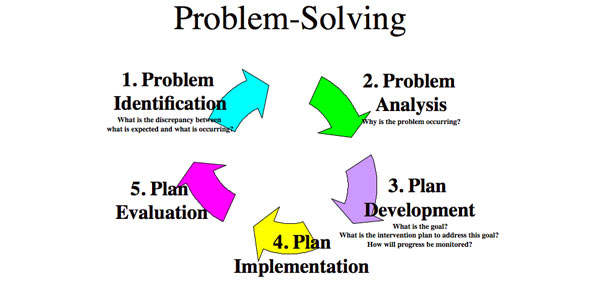# Solving Algebraic Word Problems

10 Questions | Attempts: 754
ShareSettings• 1.
5a + 2 + 7
• 2.
The perimeter of a pool table is 30 feet. The table is twice as long as it is wide. What is the length of the pool table?Write an equation for this situation. Then solve.
• 3.
-2d + 7 = 22 Solve the equation.
• 4.
Chose the following answer that is correct.
• A.

2n + 3n + 2 = -41 n= 8.6

• B.

4(2a +2) - 17 = 15 a= 3

• C.

-w + 4(w+3) = -12 w= 0

• 5.
To mail a first class letter, the U.S. Postal Service charges are \$.34 for the first ounce and \$.21 for each additional ounce. It costs \$1.18 to mail your letter. How many ounces does your letter weigh?
• A.

4 ounces

• B.

6 ounces

• C.

3.2 ounces

• 6.
9 = 5 + 4r Solve for r.
• A.

R = 1

• B.

R = 1.47

• 7.
4a + 1 = 13 Solve for a.
• A.

A = -3

• B.

A = 3

• 8.
Chose the first step you would do in the following equation. 24 - x = -16
• A.

• B.

Subtracting 24 to both sides would be the first step.

• C.

Adding 24 to both sides would be the first step.

• D.

Adding 16 to both sides would be the first step.

• 9.
Solve this equation 4s - 13 = 51
• A.

S = 16

• B.

S = 9.5

• 10.
2x + 23 = 49
• A.

X = 28

• B.

X = 13

## Related TopicsBack to top
×

Wait!
Here's an interesting quiz for you.